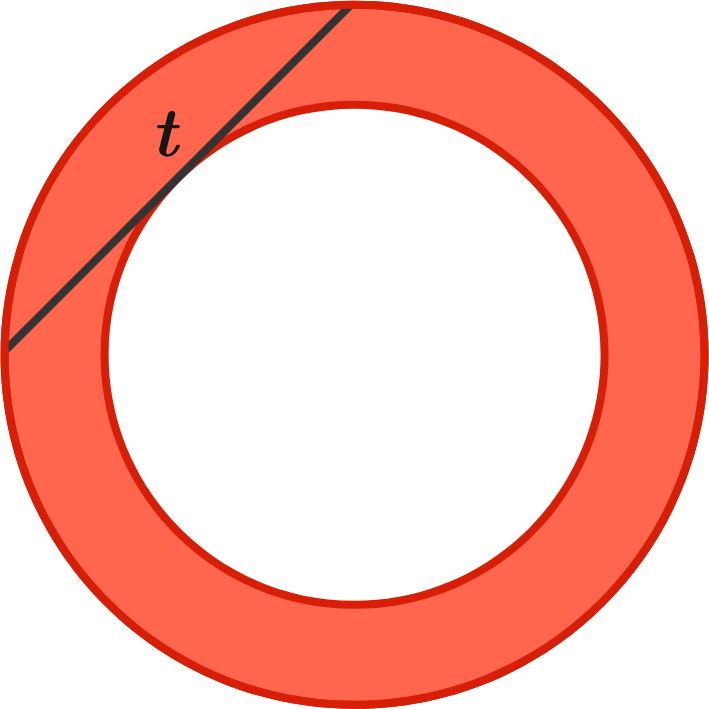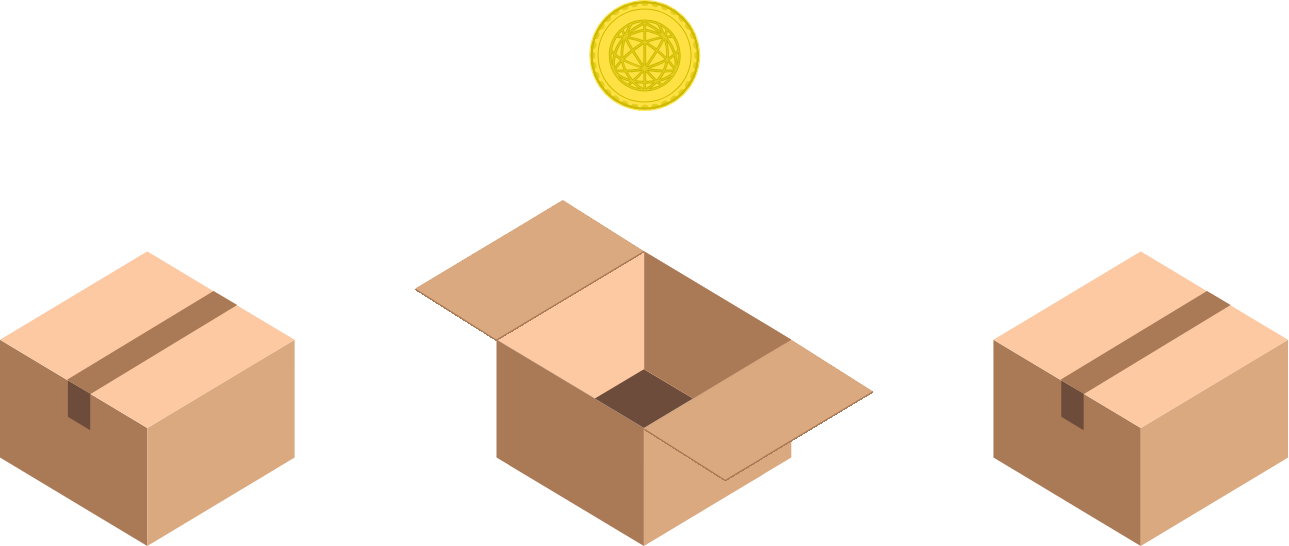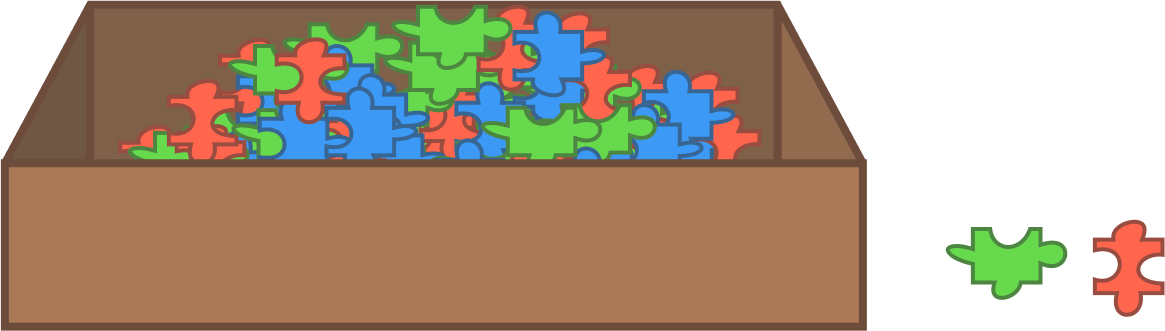# Problems of the Week

Contribute a problem

# 2018-11-19 IntermediateThis annulus has been formed from two concentric circles. A chord of length $t$ of the larger circle is then drawn tangent to the inner circle.

If you only know the value of $t,$ can you calculate the area of the annulus?

French mathematician Joseph Bertrand posed the following problem in 1889:

You have 3 identical boxes, each containing 2 coins: the first box 2 gold coins, the second box 2 silver coins, and the third box 1 gold and 1 silver coin.

Your friend shuffles the boxes at random. Then, you choose a box and pull a coin out of it, and it's gold.

What is the probability that the other coin in the same box is also a gold coin?I have a square puzzle consisting of $10 \times 10 = 100$ pieces.

If I pick two pieces at random, what is the probability that they fit together, i.e. they lie next to each other in the puzzle?

If the probability can be written as $\frac ab$ with coprime positive integers $a$ and $b,$ give your answer as $a+b.$Details and Assumptions:

• Every piece is equally likely to be picked. I don't look whether the first piece has a straight edge or similar tricks.
• Every piece only fits together with its neighboring pieces, and there is a unique solution to the puzzle.Four congruent semicircles are packed into a square.

What proportion of the square is filled?

The sum of the ages of my five nieces is 47. Their ages are positive integers, and any two of them have a common divisor greater than 1.

How old is the eldest?

×